# vs.eyeandcontacts.com

## Chapter 4 Practical Geometry Exercise 4.5

Draw the following.
Question 1: The square READ with RE = 5.1 cm.

Given
RE = 5.1 cm

Rough figure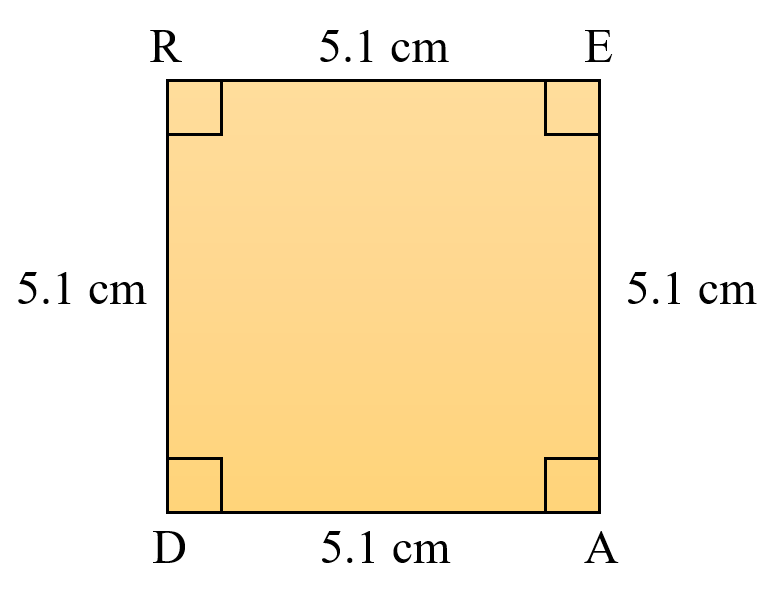Actual figure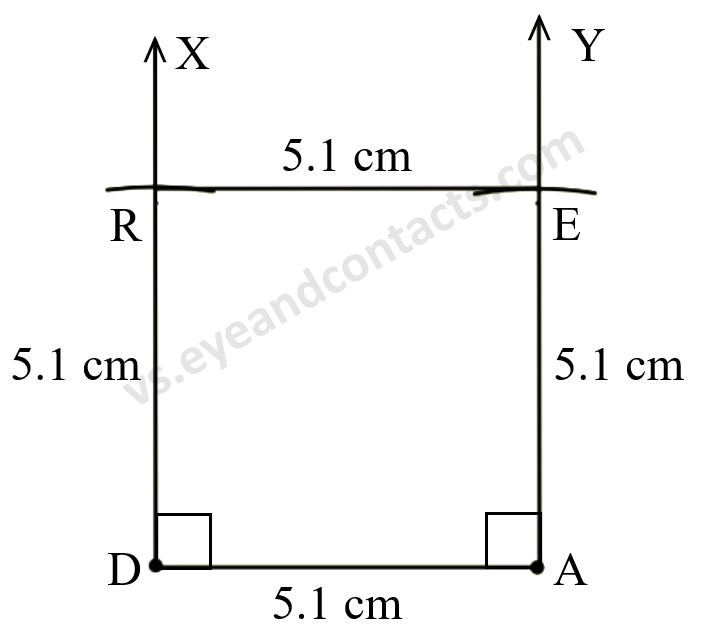Steps of Construction
1. Draw line segment DA = 5.1 cm.
2. Make ∠XDA = 90°.
4. With D as centre, radius 5.1 cm draw an arc to cut ray XD at R.
5. With A as centre, radius 5.1 cm draw an arc to cut ray YA at E.
6. Join R and E.

Question 2: A rhombus whose diagonals are 5.2 cm and 6.4 cm long.

Given
Rhombus
Diagonals = 5.2 cm, 6.4 cm

Rough figure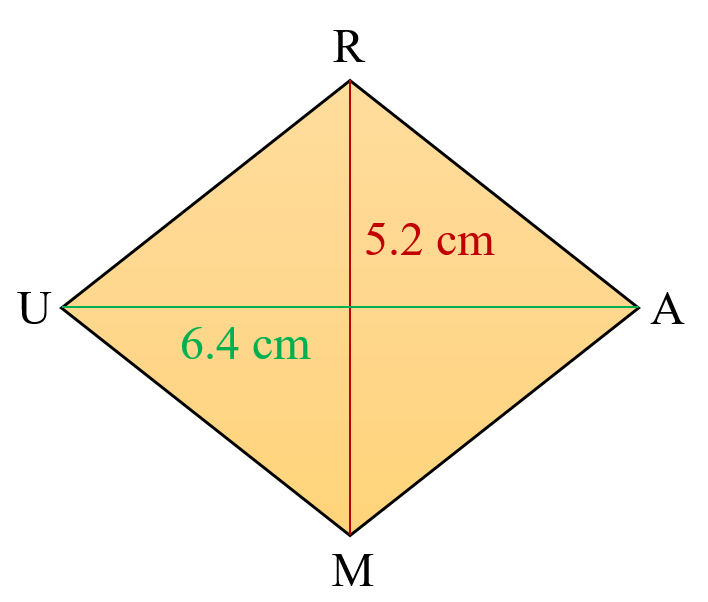Actual figure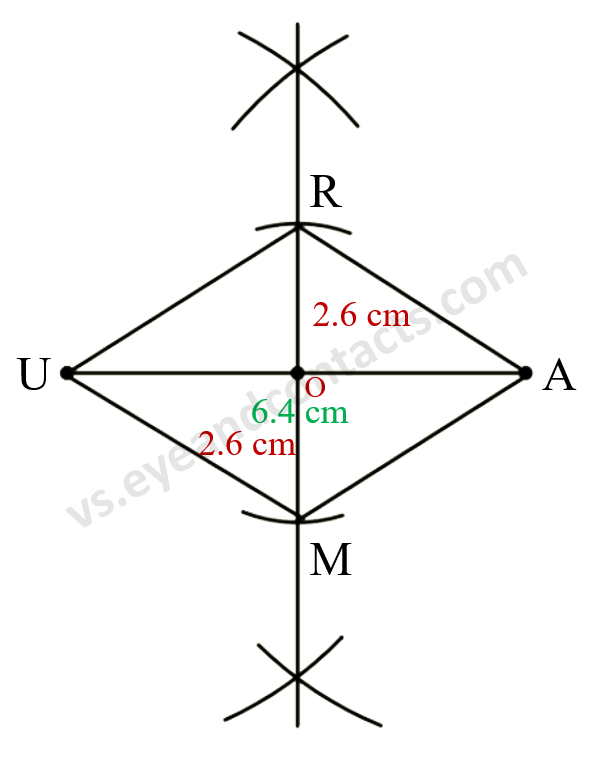Steps of Construction
1. Draw line segment UA = 6.4 cm.
2. Draw the perpendicular bisector of UA at O. (O is the midpoint of UA).
3. With O as centre, radius 2.6 cm draw arcs on either sides to intersect the perpendicular bisector at R and M.
4. Join RU, RA, UM, AM.
5. RAMU is the required rhombus.

Question 3: A rectangle with adjacent sides of lengths 5 cm and 4 cm.

Given
Rectangle
Length = 5 cm

Rough figure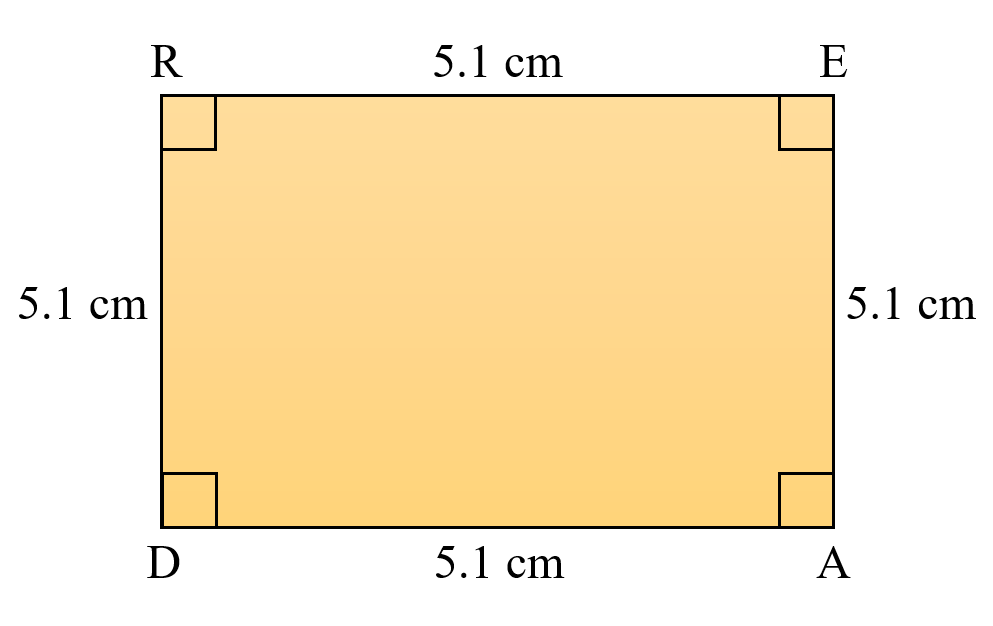Actual figure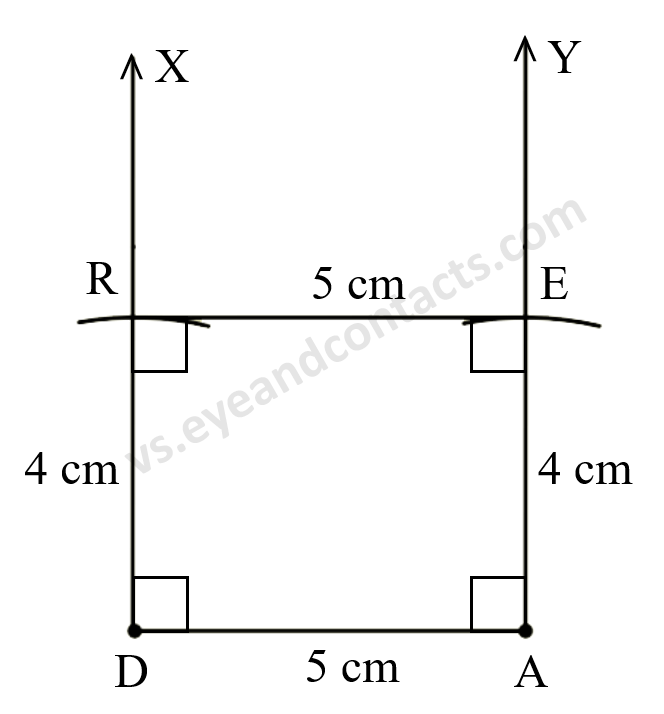Steps of Construction
1. Draw line segment DA = 5 cm.
2. Make ∠XDA = 90°.
4. With D as centre, radius 4 cm draw an arc to cut ray XD at R.
5. With A as centre, radius 4 cm draw an arc to cut ray YA at E.
6. Join R and E.
7. READ is the required rectangle.

Question 4: A parallelogram OKAY where OK = 5.5 cm and KA = 4.2 cm.

To draw any quadrilateral, five measurements would be required. This would be possible when
• When four sides and one diagonal are given.
• When two diagonals and three sides are given.
• When two adjacent sides and three angles are given.
• When three sides and two included angles are given.
• When other special properties are known.
But in parallelogram OKAY only 4 measures are given (opposite sides are equal). So any 1 measurement can be useful for making this parallelogram. It may include angle between the sides or one diagonal. Therefore, this parallelogram can’t be drawn.